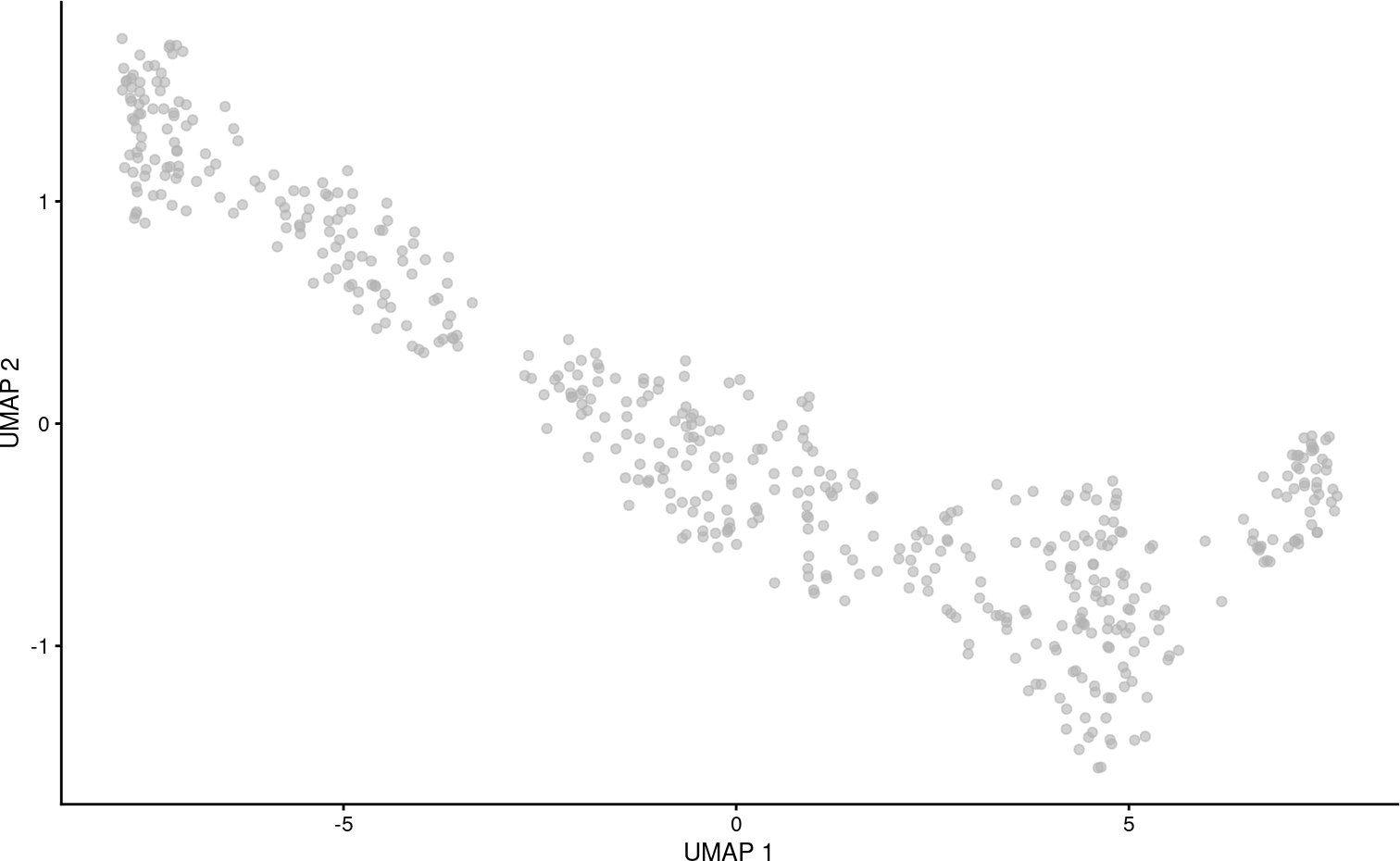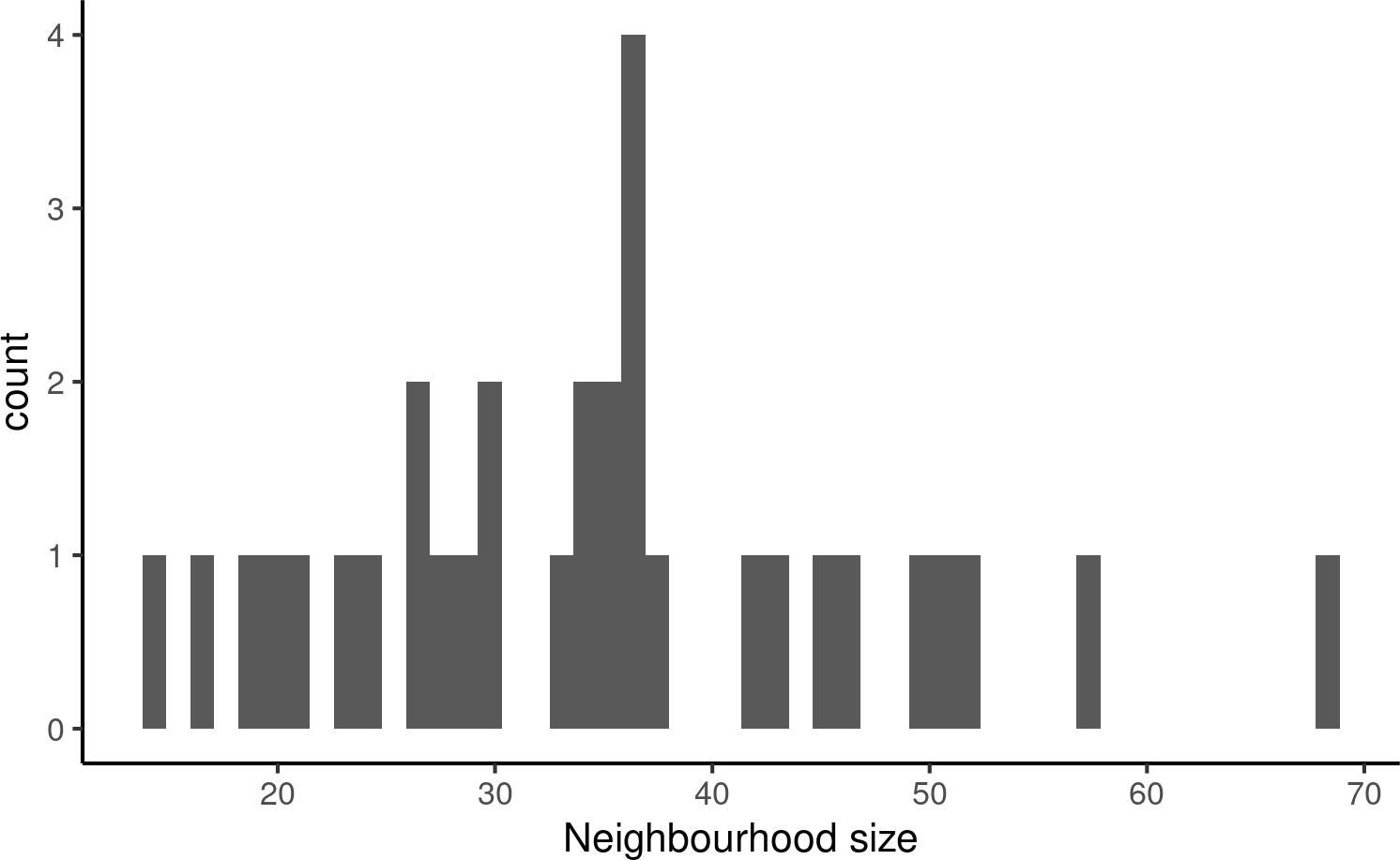library(miloR)
library(SingleCellExperiment)
library(scater)
library(scran)
library(dplyr)
library(patchwork)

# 1 Introduction

Milo is a tool for analysis of complex single cell datasets generated from replicated multi-condition experiments, which detects changes in composition between conditions. While differential abundance (DA) is commonly quantified in discrete cell clusters, Milo uses partially overlapping neighbourhoods of cells on a KNN graph. Starting from a graph that faithfully recapitulates the biology of the cell population, Milo analysis consists of 3 steps:

1. Sampling of representative neighbourhoods
2. Testing for differential abundance of conditions in all neighbourhoods
3. Accounting for multiple hypothesis testing using a weighted FDR procedure that accounts for the overlap of neighbourhoods

In this vignette we will elaborate on how these steps are implemented in the miloR package.

For this demo we will use a synthetic dataset simulating a developmental trajectory, generated using dyntoy.

data("sim_trajectory", package = "miloR")

## Extract SingleCellExperiment object
traj_sce <- sim_trajectory[['SCE']]

## Extract sample metadata to use for testing
traj_meta <- sim_trajectory[["meta"]]

colData(traj_sce) <- DataFrame(traj_meta)

# 3 Pre-processing

For DA analysis we need to construct an undirected KNN graph of single-cells. Standard single-cell analysis pipelines usually do this from distances in PCA. We normalize and calculate principal components using scater. I also run UMAP for visualization purposes.

logcounts(traj_sce) <- log(counts(traj_sce) + 1)
traj_sce <- runPCA(traj_sce, ncomponents=30)
traj_sce <- runUMAP(traj_sce)

plotUMAP(traj_sce)# 4 Create a Milo object

For differential abundance analysis on graph neighbourhoods we first construct a Milo object. This extends the SingleCellExperiment class to store information about neighbourhoods on the KNN graph.

## 4.1 From SingleCellExperiment object

The Milo constructor takes as input a SingleCellExperiment object.

traj_milo <- Milo(traj_sce)
reducedDim(traj_milo, "UMAP") <- reducedDim(traj_sce, "UMAP")

traj_milo
## class: Milo
## dim: 500 500
## assays(2): counts logcounts
## rownames(500): G1 G2 ... G499 G500
## rowData names(0):
## colnames: NULL
## colData names(5): cell_id group_id Condition Replicate Sample
## reducedDimNames(2): PCA UMAP
## mainExpName: NULL
## altExpNames(0):
## nhoods dimensions(2): 1 1
## nhoodCounts dimensions(2): 1 1
## nhoodDistances dimension(1): 0
## graph names(0):
## nhoodIndex names(1): 0
## nhoodExpression dimension(2): 1 1
## nhoodReducedDim names(0):
## nhoodGraph names(0):
## nhoodAdjacency dimension(2): 1 1

## 4.2 From AnnData object (.h5ad)

We can use the zellkonverter package to make a SingleCellExperiment object from an AnnData object stored as h5ad file.

library(zellkonverter)

# Obtaining an example H5AD file.
package = "zellkonverter")

example_h5ad_milo <- Milo(example_h5ad_sce)

## 4.3 From Seurat object

The Seurat package includes a converter to SingleCellExperiment.

library(Seurat)
data("pbmc_small")
pbmc_small_sce <- as.SingleCellExperiment(pbmc_small)
pbmc_small_milo <- Milo(pbmc_small_sce)

# 5 Construct KNN graph

We need to add the KNN graph to the Milo object. This is stored in the graph slot, in igraph format. The miloR package includes functionality to build and store the graph from the PCA dimensions stored in the reducedDim slot.

traj_milo <- buildGraph(traj_milo, k = 10, d = 30)
## Constructing kNN graph with k:10

In progress: we are perfecting the functionality to add a precomputed KNN graph (for example constructed with Seurat or scanpy) to the graph slot using the adjacency matrix.

# 6 1. Defining representative neighbourhoods

We define the neighbourhood of a cell, the index, as the group of cells connected by an edge in the KNN graph to the index cell. For efficiency, we don’t test for DA in the neighbourhood of every cell, but we sample as indices a subset of representative cells, using a KNN sampling algorithm used by Gut et al. 2015.

For sampling you need to define a few parameters:

• prop: the proportion of cells to randomly sample to start with (usually 0.1 - 0.2 is sufficient)
• k: the k to use for KNN refinement (we recommend using the same k used for KNN graph building)
• d: the number of reduced dimensions to use for KNN refinement (we recommend using the same d used for KNN graph building)
• refined indicated whether you want to use the sampling refinement algorithm, or just pick cells at random. The default and recommended way to go is to use refinement. The only situation in which you might consider using random instead, is if you have batch corrected your data with a graph based correction algorithm, such as BBKNN, but the results of DA testing will be suboptimal.
traj_milo <- makeNhoods(traj_milo, prop = 0.1, k = 10, d=30, refined = TRUE)
## Checking valid object
## Running refined sampling with reduced_dim
## Warning in .refined_sampling(random_vertices, X_reduced_dims, k): Rownames not
## set on reducedDims - setting to row indices

Once we have defined neighbourhoods, it’s good to take a look at how big the neighbourhoods are (i.e. how many cells form each neighbourhood). This affects the power of DA testing. We can check this out using the plotNhoodSizeHist function. Empirically, we found it’s best to have a distribution peaking between 50 and 100. Otherwise you might consider rerunning makeNhoods increasing k and/or prop (here the distribution looks ludicrous because it’s a small dataset).

plotNhoodSizeHist(traj_milo)# 7 Counting cells in neighbourhoods

Now we have to count how many cells from each sample are in each neighbourhood. We need to use the cell metadata and specify which column contains the sample information.

traj_milo <- countCells(traj_milo, meta.data = data.frame(colData(traj_milo)), samples="Sample")
## Checking meta.data validity
## Counting cells in neighbourhoods

This adds to the Milo object a n \times m matrix, where n is the number of neighbourhoods and $$m$$ is the number of experimental samples. Values indicate the number of cells from each sample counted in a neighbourhood. This count matrix will be used for DA testing.

head(nhoodCounts(traj_milo))
## 6 x 6 sparse Matrix of class "dgCMatrix"
##   B_R1 A_R1 A_R2 B_R2 B_R3 A_R3
## 1    5    3    3    5    6    8
## 2    2    1    .    3   10    3
## 3    6    6    4    5    9    8
## 4   13    5    3   14   16    1
## 5   11    .    1    4   16    2
## 6   10    4    1    8   18    1

# 8 Differential abundance testing

Now we are all set to test for differential abundance in neighbourhoods. We implement this hypothesis testing in a generalized linear model (GLM) framework, specifically using the Negative Binomial GLM implementation in edgeR.

We first need to think about our experimental design. The design matrix should match samples to a condition of interest. In this case the Condition is the covariate we are going to test for.

traj_design <- data.frame(colData(traj_milo))[,c("Sample", "Condition")]
traj_design <- distinct(traj_design)
rownames(traj_design) <- traj_design$Sample ## Reorder rownames to match columns of nhoodCounts(milo) traj_design <- traj_design[colnames(nhoodCounts(traj_milo)), , drop=FALSE] traj_design ## Sample Condition ## B_R1 B_R1 B ## A_R1 A_R1 A ## A_R2 A_R2 A ## B_R2 B_R2 B ## B_R3 B_R3 B ## A_R3 A_R3 A Milo uses an adaptation of the Spatial FDR correction introduced by cydar, which accounts for the overlap between neighbourhoods. Specifically, each hypothesis test P-value is weighted by the reciprocal of the kth nearest neighbour distance. To use this statistic we first need to store the distances between nearest neighbors in the Milo object. traj_milo <- calcNhoodDistance(traj_milo, d=30) Now we can do the test, explicitly defining our experimental design. rownames(traj_design) <- traj_design$Sample
da_results <- testNhoods(traj_milo, design = ~ Condition, design.df = traj_design)
## Using TMM normalisation
## Performing spatial FDR correction withk-distance weighting

This calculates a Fold-change and corrected P-value for each neighbourhood, which indicates whether there is significant differential abundance between conditions.

da_results %>%
arrange(- SpatialFDR) %>%
head() 
##          logFC   logCPM          F    PValue       FDR Nhood SpatialFDR
## 30  0.06689997 15.75163 0.02255352 0.8808603 0.8808603    30  0.8808603
## 27  0.15089653 14.70102 0.05209013 0.8378946 0.8649234    27  0.8648400
## 28 -0.20816385 15.17910 0.12719730 0.7219431 0.7700726    28  0.7662347
## 22 -0.48420164 15.47867 1.51595720 0.3168746 0.3496547    22  0.3480570
## 2   0.88631939 14.84779 1.36590092 0.2446894 0.2796451     2  0.2783758
## 18 -0.66338439 15.25127 1.52835194 0.2186237 0.2591096    18  0.2572268

# 9 Visualize neighbourhoods displaying DA

To visualize DA results, we build an abstracted graph of neighbourhoods that we can superimpose on the single-cell embedding.

traj_milo <- buildNhoodGraph(traj_milo)

plotUMAP(traj_milo) + plotNhoodGraphDA(traj_milo, da_results, alpha=0.05) +
plot_layout(guides="collect")